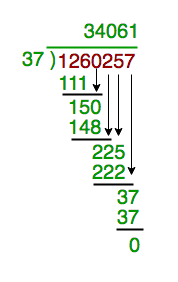Related Articles

# Divide large number represented as string

• Difficulty Level : Medium
• Last Updated : 29 Apr, 2021

Given a large number (represented as a string) which has to divide by another number (represented as int data type). The large number can be very large which does not even fit in long long in C++. The task is to find the division of these numbers.
Examples:

```Input : number  = 1260257
divisor = 37
Output : 34061
(See below diagram)

Input : number  = 12313413534672234
divisor = 754
Output : 16330787181262

Input : number  = 1248163264128256512
divisor = 125
Output : 9985306113026052```

We have already discussed Multiply Large Numbers represented as Strings.
We use basic school mathematics as shown in below example.As the dividend and result can be very large we store them in string. We first take digits which are divisible by number. After this take each digit and store result in string.

## C++

 `// C++ program to implement division with large``// number``#include ``using` `namespace` `std;` `// A function to perform division of large numbers``string longDivision(string number, ``int` `divisor)``{``    ``// As result can be very large store it in string``    ``string ans;` `    ``// Find prefix of number that is larger``    ``// than divisor.``    ``int` `idx = 0;``    ``int` `temp = number[idx] - ``'0'``;``    ``while` `(temp < divisor)``        ``temp = temp * 10 + (number[++idx] - ``'0'``);` `    ``// Repeatedly divide divisor with temp. After``    ``// every division, update temp to include one``    ``// more digit.``    ``while` `(number.size() > idx) {``        ``// Store result in answer i.e. temp / divisor``        ``ans += (temp / divisor) + ``'0'``;` `        ``// Take next digit of number``        ``temp = (temp % divisor) * 10 + number[++idx] - ``'0'``;``    ``}` `    ``// If divisor is greater than number``    ``if` `(ans.length() == 0)``        ``return` `"0"``;` `    ``// else return ans``    ``return` `ans;``}` `// Driver program to test longDivison()``int` `main()``{``    ``string number = ``"1248163264128256512"``;``    ``int` `divisor = 125;``    ``cout << longDivision(number, divisor);``    ``return` `0;``}`

## Java

 `// Java program to implement division``// with large number``class` `GFG {``    ``public` `static` `String longDivision(``        ``String number,``        ``int` `divisor)``    ``{` `        ``// As result can be very``        ``// large store it in string``        ``// but since we need to modify``        ``// it very often so using``        ``// string builder``        ``StringBuilder result``            ``= ``new` `StringBuilder();` `        ``// We will be iterating``        ``// the dividend so converting``        ``// it to char array``        ``char``[] dividend``            ``= number.toCharArray();` `        ``// Initially the carry``        ``// would be zero``        ``int` `carry = ``0``;` `        ``// Iterate the dividend``        ``for` `(``            ``int` `i = ``0``;``            ``i < dividend.length; i++) {``            ``// Prepare the number to``            ``// be divided``            ``int` `x``                ``= carry * ``10``                  ``+ Character.getNumericValue(``                        ``dividend[i]);` `            ``// Append the result with``            ``// partial quotient``            ``result.append(x / divisor);` `            ``// Prepare the carry for``            ``// the next Iteration``            ``carry = x % divisor;``        ``}` `        ``// Remove any leading zeros``        ``for` `(``            ``int` `i = ``0``;``            ``i < result.length(); i++) {``            ``if` `(``                ``result.charAt(i) != ``'0'``) {``                ``// Return the result``                ``return` `result.substring(i);``            ``}``        ``}``        ``// Return empty string``        ``// if number is empty``        ``return` `""``;``    ``}` `    ``// Driver code``    ``public` `static` `void` `main(``        ``String[] args)``    ``{``        ``String number``            ``= ``"1248163264128256512"``;``        ``int` `divisor = ``125``;``        ``System.out.println(``            ``longDivision(``                ``number, divisor));``    ``}``}` `// This code is contributed by Saurabh321Gupta.`

## Python3

 `# Python3 program to implement division``# with large number``import` `math` `# A function to perform division of``# large numbers``def` `longDivision(number, divisor):` `    ``# As result can be very large``    ``# store it in string``    ``ans ``=` `"";``    ` `    ``# Find prefix of number that``    ``# is larger than divisor.``    ``idx ``=` `0``;``    ``temp ``=` `ord``(number[idx]) ``-` `ord``(``'0'``);``    ``while` `(temp < divisor):``        ``temp ``=` `(temp ``*` `10` `+` `ord``(number[idx ``+` `1``]) ``-``                            ``ord``(``'0'``));``        ``idx ``+``=` `1``;``    ` `    ``idx ``+``=` `1``;` `    ``# Repeatedly divide divisor with temp.``    ``# After every division, update temp to``    ``# include one more digit.``    ``while` `((``len``(number)) > idx):``        ` `        ``# Store result in answer i.e. temp / divisor``        ``ans ``+``=` `chr``(math.floor(temp ``/``/` `divisor) ``+` `ord``(``'0'``));``        ` `        ``# Take next digit of number``        ``temp ``=` `((temp ``%` `divisor) ``*` `10` `+` `ord``(number[idx]) ``-``                                        ``ord``(``'0'``));``        ``idx ``+``=` `1``;` `    ``ans ``+``=` `chr``(math.floor(temp ``/``/` `divisor) ``+` `ord``(``'0'``));``    ` `    ``# If divisor is greater than number``    ``if` `(``len``(ans) ``=``=` `0``):``        ``return` `"0"``;``    ` `    ``# else return ans``    ``return` `ans;` `# Driver Code``number ``=` `"1248163264128256512"``;``divisor ``=` `125``;``print``(longDivision(number, divisor));``    ` `# This code is contributed by mits`

## C#

 `// C# program to implement division``// with large number``using` `System;` `class` `GFG {` `    ``// A function to perform division of large numbers``    ``static` `string` `longDivision(``string` `number, ``int` `divisor)``    ``{``        ``// As result can be very large store it in string``        ``string` `ans = ``""``;` `        ``// Find prefix of number that is larger``        ``// than divisor.``        ``int` `idx = 0;``        ``int` `temp = (``int``)(number[idx] - ``'0'``);``        ``while` `(temp < divisor) {``            ``temp = temp * 10 + (``int``)(number[idx + 1] - ``'0'``);``            ``idx++;``        ``}``        ``++idx;` `        ``// Repeatedly divide divisor with temp. After``        ``// every division, update temp to include one``        ``// more digit.``        ``while` `(number.Length > idx) {``            ``// Store result in answer i.e. temp / divisor``            ``ans += (``char``)(temp / divisor + ``'0'``);` `            ``// Take next digit of number``            ``temp = (temp % divisor) * 10 + (``int``)(number[idx] - ``'0'``);``            ``idx++;``        ``}``        ``ans += (``char``)(temp / divisor + ``'0'``);` `        ``// If divisor is greater than number``        ``if` `(ans.Length == 0)``            ``return` `"0"``;` `        ``// else return ans``        ``return` `ans;``    ``}` `    ``// Driver code``    ``static` `void` `Main()``    ``{``        ``string` `number = ``"1248163264128256512"``;``        ``int` `divisor = 125;``        ``Console.WriteLine(longDivision(number, divisor));``    ``}``}` `// This code is contributed by mits`

## PHP

 ` ``\$idx``)``    ``{``        ``// Store result in answer i.e. temp / divisor``        ``\$ans` `.= ``chr``((int)(``\$temp` `/ ``\$divisor``) + 48);``        ` `        ``// Take next digit of number``        ``\$temp` `= (``\$temp` `% ``\$divisor``) * 10 +``             ``ord(``\$number``[``\$idx``]) - 48;``        ``++``\$idx``;``    ``}``    ``\$ans` `.= ``chr``((int)(``\$temp` `/ ``\$divisor``) + 48);``    ` `    ``// If divisor is greater than number``    ``if` `(``strlen``(``\$ans``) == 0)``        ``return` `"0"``;``    ` `    ``// else return ans``    ``return` `\$ans``;``}` `// Driver Code``\$number` `= ``"1248163264128256512"``;``\$divisor` `= 125;``print``(longDivision(``\$number``, ``\$divisor``));` `// This code is contributed by mits``?>`

## Javascript

 ``

Output:

`9985306113026052`

This article is contributed by nuclode. If you like GeeksforGeeks and would like to contribute, you can also write an article using contribute.geeksforgeeks.org or mail your article to contribute@geeksforgeeks.org. See your article appearing on the GeeksforGeeks main page and help other Geeks.